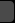## Archive for the ‘mathematics’ category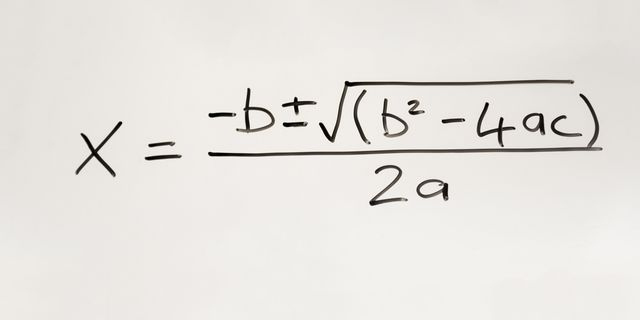Quadratic equations are polynomials, meaning strings of math terms. An expression like “x + 4” is a polynomial. They can have one or many variables in any combination, and the magnitude of them is decided by what power the variables are taken to. So x + 4 is an expression describing a straight line, but (x + 4)² is a curve. Since a line crosses just once through any particular latitude or longitude, its solution is just one value. If you have x², that means two root values, in a shape like a circle or arc that makes two crossings.Evolutionary stories like the grandmother hypothesis are easy to construct from mathematical models, but how well do they reflect reality?An international group of scientists, including Andrey Savelyev, associate professor of the Institute of Physical and Mathematical Sciences and Information Technologies of the IKBFU, has improved a computer program that helps simulate the behavior of photons when interacting with hydrogen spilled in intergalactic space. Results are published in the scientific journal Monthly Notices of the Royal Astronomical Society.

Andrey Saveliev states, “In the Universe there are extragalactic objects such as blazars, which very intensively generate a powerful gamma-ray flux, part of photons from this stream reaches the Earth, as they say, directly, and part are converted along the way into electrons, then again converted into photons and only then get to us. The problem here is that say that a certain number of photons should reach the Earth, and in fact it is much less.”

Scientists, according to Andrey Savelyev, today have two versions of why this happens. The first is that a , after being converted into an electron (and this, as is known, in contrast to a neutral photon, a charged particle) falls into a , deviates from its path and does not reach the Earth, even after being transformed again into the photon.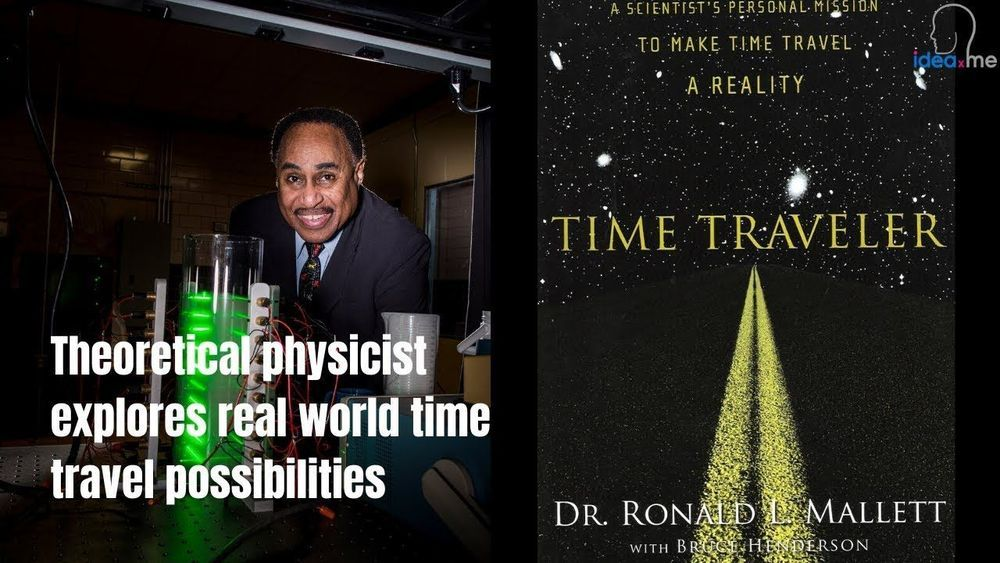Ira Pastor, ideaXme exponential health ambassador, interviews Dr. Ronald Mallett, Professor Emeritus, Theoretical Physics, Department of Physics at the University of Connecticut.Scientists hope that the future of gravitational wave detection will allow them to directly observe a mysterious kind of black hole.

Gravitational wave detectors have seen direct evidence of black holes with roughly the mass of giant stars, while the Event Horizon Telescope produced an image of a supermassive black hole billions of times the mass of our Sun. But in the middle are intermediate-mass black holes, or IMBHs, which weigh between 100 and 100,000 times the mass of the Sun and have yet to be directly observed. Researchers hope that their new mathematical work will “pave the way” for future research into these black holes using gravitational wave detectors, according to the paper published today in Nature Astronomy.Three physicists stumbled across an unexpected relationship between some of the most ubiquitous objects in math.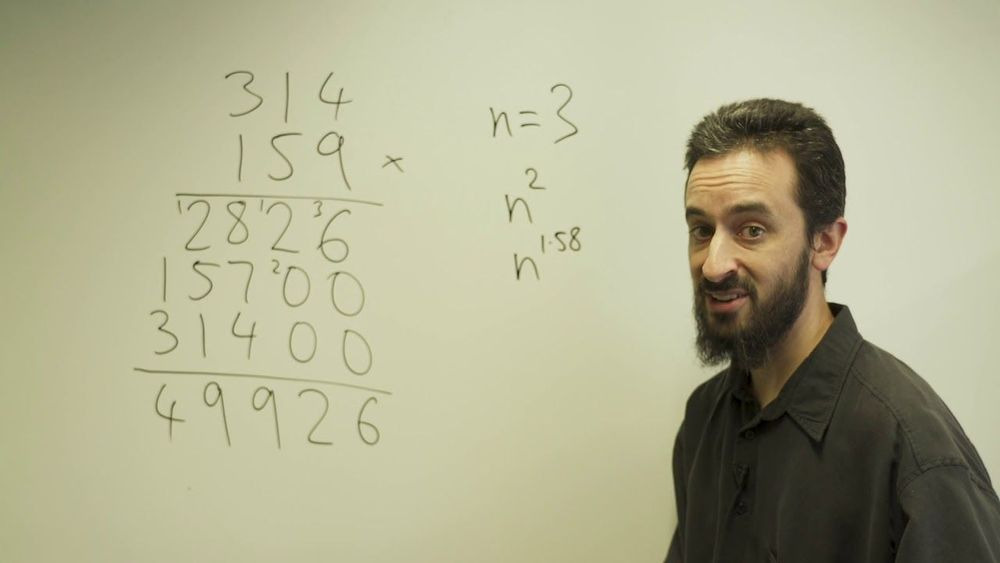A pair of mathematicians from Australia and France have devised an alternative way to multiply numbers together, while solving an algorithmic puzzle that has perplexed some of the greatest math minds for almost half a century.

For most of us, the way we multiply relatively small numbers is by remembering our times tables – an incredibly handy aid first pioneered by the Babylonians some 4,000 years ago.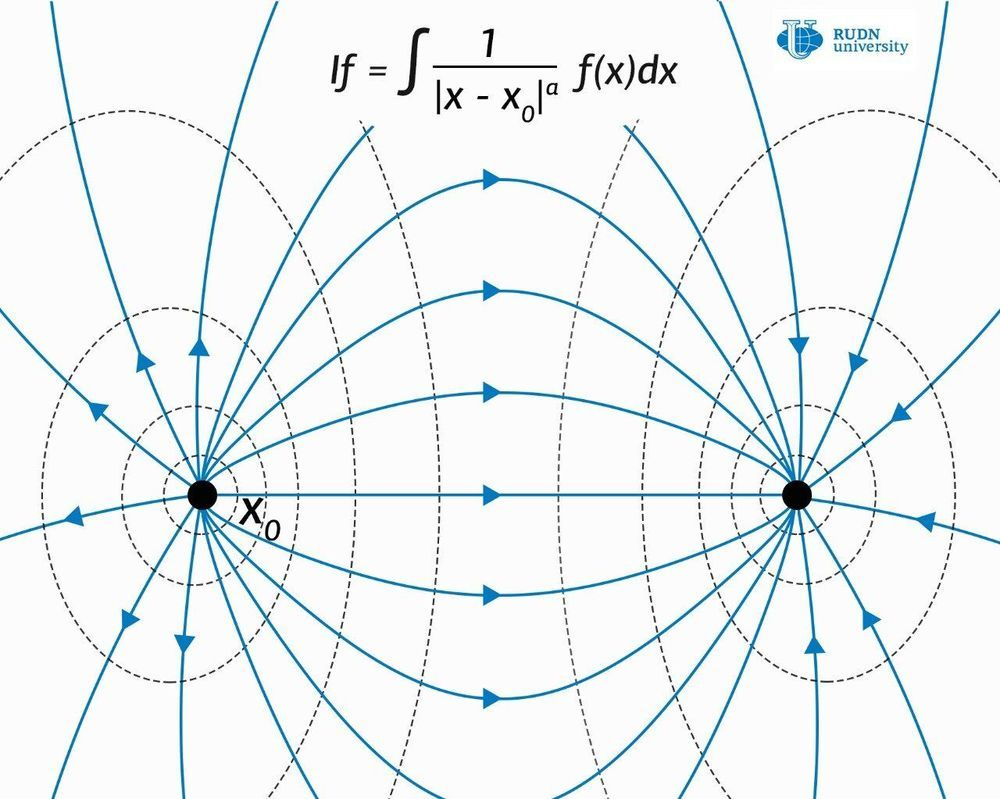RUDN University mathematicians have proven the Hardy-Littlewood-Sobolev (HLS) inequalities for the class of generalized Riesz potentials. These results extend the scope of these potentials in mathematics and physics because the main tools for working with such potentials are based on HLS inequalities. New mathematical tools can greatly simplify calculations in quantum mechanics and other fields of physics. The results of the study are published in the journal Mathematical Notes.

Modern physics describes the world in terms of fields and their potentials—that is, the values of the field at each point. But the physical quantities that we can measure are forces and accelerations, that is, derivatives of the second-order of the potential of the corresponding field. The problem of reconstructing the field configuration with the available values of forces and accelerations observed in experiments is complex and not always analytically solvable. Differentiation operations in multidimensional space—operators are usually used to describe the correlation between the potential of the field and the forces. In particular, electromagnetic and gravitational interactions are described in the language of operators.

Since the potential of the field can be determined up to a constant value, for the convenience of calculations, the initial value of the potential is taken at some point in multidimensional space, or on the border of any spatial area. But in some cases, mathematical models of such fields lead to a singularity, that is, at some points the value of the field becomes infinite, and therefore loses its physical meaning.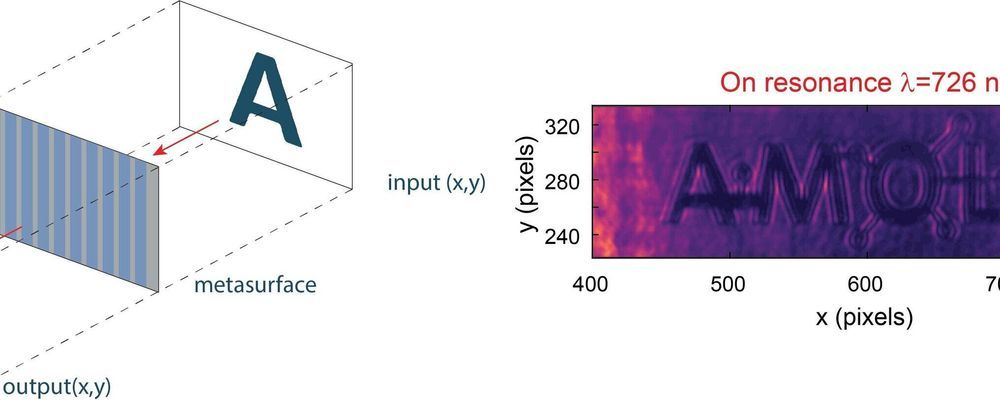AMOLF researchers and their collaborators from the Advanced Science Research Center (ASRC/CUNY) in New York have created a nanostructured surface capable of performing on-the-fly mathematical operations on an input image. This discovery could boost the speed of existing imaging processing techniques and lower energy usage. The work enables ultrafast object detection and augmented reality applications. The researchers publish their results today in the journal Nano Letters.

Image processing is at the core of several rapidly growing technologies, such as augmented reality, autonomous driving and more general object recognition. But how does a computer find and recognize an object? The initial step is to understand where its boundaries are, hence edge detection in an image becomes the starting point for image recognition. Edge detection is typically performed digitally using integrated implying fundamental speed limitations and high energy consumption, or in an analog fashion which requires bulky optics.In 1884, a schoolmaster and theologian named Edwin Abbott wrote a novella called Flatland, which tells the story of a world populated by sentient two-dimensional shapes. While intended as a satire of rigid Victorian social norms, Flatland has long fascinated mathematicians and physicists and served as the setting for many a thought experiment.

One such thought experiment: How can be controlled in two dimensions?

When a wave of light is confined on a two-dimensional plane by certain materials, it becomes something known as a —a particle that blurs the distinction between light and matter. Polaritons have exciting implications for the future of optical circuits because, unlike electronic integrated circuits, integrated optics is difficult to miniaturize with commonly used materials. Polaritons allow light to be tightly confined to the nanoscale, even potentially to the thickness of a few atoms.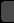Page 1 of 3012345678Last